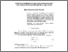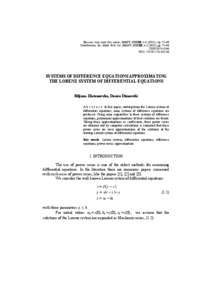# Systems of difference equations approximating the Lorenz system of differential equations

Zlatanovska, Biljana and Dimovski, Donco (2012) Systems of difference equations approximating the Lorenz system of differential equations. Contributions, Sec. Math. Tech. Sci., XXXIII (1-2). ISSN 0351–3246Preview
Text
Prilozi-33-1-2-2012-05-Dimovskii.pdfView Item